π-calculus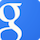π-calculus

## Tag #π-calculus

### 83 papers:POPL-2019-LagoVMY #fault #runtime
Intersection types and runtime errors in the pi-calculus (UDL, MdV, DM, AY), p. 29.ESOP-2019-SakayoriT #category theory
A Categorical Model of an i/o-typed π-calculus (KS, TT), pp. 640–667.CASE-2016-CampbellTLPOF #approach #concurrent #configuration management #modelling
Modeling concurrency and reconfiguration in vehicular systems: A π-calculus approach (JC, CET, PL0, TPP, ÜÖ, GEF), pp. 523–530.ESOP-2016-DOsualdoO #communication #on the
On Hierarchical Communication Topologies in the π-calculus (ED, CHLO), pp. 149–175.TLCA-2015-Assaf
Conservativity of Embeddings in the λ π Calculus Modulo Rewriting (AA0), pp. 31–44.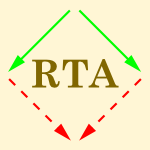RTA-TLCA-2014-HondaYB #interactive #process
Process Types as a Descriptive Tool for Interaction — Control and the π-Calculus (KH, NY, MB), pp. 1–20.FoSSaCS-2014-Padovani #linear #re-engineering #recursion
Type Reconstruction for the Linear π-Calculus with Composite and Equi-Recursive Types (LP), pp. 88–102.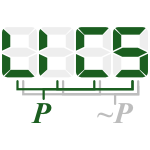LICS-CSL-2014-Padovani #concurrent #linear
Deadlock and lock freedom in the linear π-calculus (LP), p. 10.LATA-2013-DengZDZ #concurrent
The Buffered π-Calculus: A Model for Concurrent Languages (XD, YZ, YD, FZ), pp. 250–261.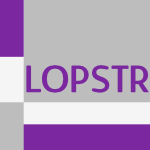LOPSTR-2013-Saeedloei #encoding #logic
A Logical Encoding of Timed π-Calculus (NS), pp. 164–182.FoSSaCS-2013-DreierELL #composition #on the #process
On Unique Decomposition of Processes in the Applied π-Calculus (JD, CE, PL, YL), pp. 50–64.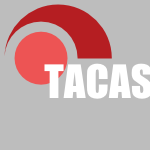TACAS-2013-MateescuS #model checking #model transformation #named
PIC2LNT: Model Transformation for Model Checking an Applied π-Calculus (RM, GS), pp. 192–198.PPDP-2012-MonjarazM #haskell
From the π-calculus to flat GHC (RM, JM), pp. 163–172.FoSSaCS-2012-CrafaVY #parallel #semantics
Event Structure Semantics of Parallel Extrusion in the π-Calculus (SC, DV, NY), pp. 225–239.BX-2011-Schmitt #higher-order
Reversible Higher Order π Calculus (AS), p. 63.QAPL-2011-SongNN #probability
A Stochastic Broadcast π-Calculus (LS, FN, BFN), pp. 74–88.IFM-2010-MateescuS
Translating π-Calculus into LOTOS NT (RM, GS), pp. 229–244.ICALP-v2-2009-AcciaiB #behaviour #infinity #safety
Deciding Safety Properties in Infinite-State π-Calculus via Behavioural Types (LA, MB), pp. 31–42.ICALP-B-2008-JohanssonPVB
Extended π-Calculi (MJ, JP, BV, JB), pp. 87–98.ESOP-2008-BuscemiM #bisimulation #concurrent #constraints
Open Bisimulation for the Concurrent Constraint π-Calculus (MGB, UM), pp. 254–268.TAP-2008-Cao #equivalence #finite #higher-order
Equivalence Checking for a Finite Higher Order π-Calculus (ZC), pp. 30–47.TLCA-2007-FaggianP #linear
Ludics is a Model for the Finitary Linear π-Calculus (CF, MP), pp. 148–162.PPDP-2007-AmadioD
Feasible reactivity in a synchronous π-calculus (RMA, FD), pp. 221–230.QAPL-2007-VaraccaY #probability
Probabilistic π-Calculus and Event Structures (DV, NY), pp. 147–166.FoSSaCS-2007-BengtsonP #formal method #logic #using
Formalising the π-Calculus Using Nominal Logic (JB, JP), pp. 63–77.FoSSaCS-2007-HirschkoffP #congruence
A Distribution Law for CCS and a New Congruence Result for the π-Calculus (DH, DP), pp. 228–242.LICS-2007-BonsangueK #logic
π-Calculus in Logical Form (MMB, AK), pp. 303–312.PPDP-2006-BundgaardS #graph
Typed polyadic π-calculus in bigraphs (MB, VS), pp. 1–12.SAC-2006-Lecca #algorithm #probability
A time-dependent extension of gillespie algorithm for biochemical stochastic π-calculus (PL), pp. 137–144.FoSSaCS-2006-Cao #bisimulation #higher-order
More on Bisimulations for Higher Order π-Calculus (ZC), pp. 63–78.TACAS-2006-YangBR #verification
Parameterized Verification of π-Calculus Systems (PY, SB, CRR), pp. 42–57.LICS-2006-PalamidessiSVV #on the #persistent
On the Expressiveness of Linearity vs Persistence in the Asychronous π-Calculus (CP, VAS, FDV, BV), pp. 59–68.VMCAI-2006-KobayashiSW #analysis #resource management
Resource Usage Analysis for the pi-Calculus (NK, KS, LW), pp. 298–312.ICALP-2005-BaldamusPV #encoding
A Fully Abstract Encoding of the π-Calculus with Data Terms (MB, JP, BV), pp. 1202–1213.IFM-2005-LamP #consistency #diagrams #sequence chart #statechart #using
Consistency Checking of Sequence Diagrams and Statechart Diagrams Using the π-Calculus (VSWL, JAP), pp. 347–365.ESOP-2005-KremerR #analysis #protocol
Analysis of an Electronic Voting Protocol in the Applied π Calculus (SK, MR), pp. 186–200.FoSSaCS-2005-JeffreyR #abstraction #polymorphism
Full Abstraction for Polymorphic π-Calculus (AJ, JR), pp. 266–281.FoSSaCS-2005-Stark #algebra #modelling
Free-Algebra Models for the π-Calculus (IS), pp. 155–169.SAC-2004-LeccaPLC #predict #probability
Predicting cell adhesion probability via the biochemical stochastic π-calculus (PL, CP, CL, GC), pp. 211–212.ESOP-2004-AbadiBF #performance
Just Fast Keying in the π Calculus (MA, BB, CF), pp. 340–354.FoSSaCS-2004-Caires #behaviour #logic
Behavioral and Spatial Observations in a Logic for the π-Calculus (LC), pp. 72–89.LICS-2004-BaldamusPV
Sπ Calculus Translated to ?--Calculus Preserving May-Tests (MB, JP, BV), pp. 22–31.ESOP-2003-Lhoussaine #distributed #type inference
Type Inference for a Distributed π-Calculus (CL), pp. 253–268.FoSSaCS-2003-BergerHY
Genericity and the π-Calculus (MB, KH, NY), pp. 103–119.VMCAI-2003-YangRS #encoding #logic #mobile #model checking #process #using
A Logical Encoding of the pi-Calculus: Model Checking Mobile Processes Using Tabled Resolution (PY, CRR, SAS), pp. 116–131.WRLA-2002-ThatiSM #execution #maude #semantics #specification #testing
An Executable Specification of Asynchronous π-Calculus Semantics and May Testing in Maude 2.0 (PT, KS, NMO), pp. 261–281.TLCA-2001-BergerHY
Sequentiality and the π-Calculus (MB, KH, NY), pp. 29–45.POPL-2001-IgarashiK #type system
A generic type system for the π-calculus (AI, NK), pp. 128–141.PPDP-2001-HepburnW #trust
Trust in the π-Calculus (MH, DW), pp. 103–114.SAS-2001-RajamaniR #behaviour
A Behavioral Module System for the π-Calculus (SKR, JR), pp. 375–394.FoSSaCS-2001-RocklHB #formal method #higher-order #induction #syntax
Higher-Order Abstract Syntax with Induction in Isabelle/HOL: Formalizing the π-Calculus and Mechanizing the Theory of Contexts (CR, DH, SB), pp. 364–378.LICS-2001-YoshidaBH #normalisation
Strong Normalisation in the π-Calculus (NY, MB, KH), pp. 311–322.ICALP-2000-HennessyR #data flow #resource management
Information Flow vs. Resource Access in the Asynchronous π-Calculus (MH, JR), pp. 415–427.FoSSaCS-2000-HerescuP #probability
Probabilistic Asynchronous π-Calculus (OMH, CP), pp. 146–160.FM-v2-1999-Feijs #modelling #using
Modelling Microsoft COM Using π-Calculus (LMGF), pp. 1343–1363.FoSSaCS-1999-RocklS #algol #concurrent #process #semantics
A π-calculus Process Semantics of Concurrent Idealised ALGOL (CR, DS), pp. 306–321.TACAS-1999-MontanariP #finite #verification
Finite State Verification for the Asynchronous π-Calculus (UM, MP), pp. 255–269.LICS-1999-BruniM #category theory
Cartesian Closed Double Categories, Their λ-Notation, and the π-Calculus (RB, UM), pp. 246–265.ICALP-1998-LiC #bisimulation #congruence
Checking Strong/Weak Bisimulation Equivalences and Observation Congruence for the π-Calculus (ZL, HC), pp. 707–718.ICALP-1998-Lin #proving
Complete Proof Systems for Observation Congruences in Finite-Control π-Calculus (HL), pp. 443–454.ICALP-1998-Sewell #distributed #type system
Global/Local Subtyping and Capability Inference for a Distributed π-calculus (PS), pp. 695–706.JICSLP-1998-Milner
The π Calculus and Its Applications (RM), pp. 3–4.ICALP-1997-PhilippouW #confluence #on the
On Confluence in the π-Calculus (AP, DW), pp. 314–324.PEPM-1997-GenglerM #partial evaluation #self
Self-Applicable Partial Evaluation for the π-Calculus (MG, MM), pp. 36–46.POPL-1997-Boudol
The π-calculus in Direct Style (GB), pp. 228–241.POPL-1997-Palamidessi #power of
Comparing the Expressive Power of the Synchronous and the Asynchronous π-calculus (CP), pp. 256–265.POPL-1997-PierceS #behaviour #equivalence #polymorphism
Behavioral Equivalence in the Polymorphic π-calculus (BCP, DS), pp. 242–255.ICLP-1997-Mahajan #concurrent #logic programming
Concurrent Logic Programming and π Calculus (SM, SR), p. 411.POPL-1996-KobayashiPT
Linearity and the π-Calculus (NK, BCP, DNT), pp. 358–371.TAPSOFT-J-1995-Sangiorgi96a #calculus
pi-Calculus, Internal Mobility, and Agent-Passing Calculi (DS), pp. 235–274.CAV-1996-PistoreS #algorithm #refinement
A Partition Refinement Algorithm for the π-Calculus (MP, DS), pp. 38–49.LICS-1996-FioreMS
A Fully-Abstract Model for the π-Calculus (MPF, EM, DS), pp. 43–54.LICS-1996-Stark #abstract domain #domain model
A Fully Abstract Domain Model for the π-Calculus (IS), pp. 36–42.FPCA-1995-HylandO #game studies
π-Calculus, Dialogue Games and PCF (JMEH, CHLO), pp. 96–107.ASF+SDF-1995-Deursen
A Simple π-Calculus Manipulation Tool (AvD), pp. 211–232.TAPSOFT-1995-Lin #bisimulation
Complete Inference Systems for Weak Bisimulation Equivalences in the pi-Calculus (HL), pp. 187–201.ICLP-1995-Hirata #correctness #haskell #proving
Proving Correctness of Translation from Moded Flat GHC to π-Calculus (KH), p. 818.ESOP-1994-Li #prolog #specification
A π-Calculus Specification of Prolog (BZL), pp. 379–393.ESOP-1994-Milner #named #visual notation
Pi-Nets: A Graphical Form of π-Calculus (RM), pp. 26–42.CAV-1994-VictorM
The Mobility Workbench — A Tool for the π-Calculus (BV, FM), pp. 428–440.POPL-1993-Gay #algorithm
A Sort Inference Algorithm for the Polyadic π-Calculus (SJG), pp. 429–438.TAPSOFT-1993-Sangiorgi #higher-order
From pi-Calculus to Higher-Order pi-Calculus - and Back (DS), pp. 151–166.ILPS-1993-Li #prolog #specification
A π-calculus Specification of Prolog (ZL), p. 680.

Bibliography of Software Language Engineering in Generated Hypertext (BibSLEIGH) is created and maintained by Dr. Vadim Zaytsev.
Hosted as a part of SLEBOK on GitHub.